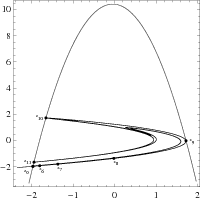The CAPD library has been used in several articles in which chaotic dynamics, bifurcations, heteroclinic/homoclinic solutions and periodic orbits were studied.

 Rigorous numerics for homoclinic tangencies Homoclinic tangecies of planar diffeomorphisms have strong dynamical consequences. In particular, the presence of such a generic homoclinic tangency implies the existence of a sequence of parameters for which attracting periodic point of arbitrary large period exists. It has been proved by Newhouse and Robinson that there is also a parameter value of the system for which infinitely many sinks coexist. Moreover, the generic homoclinic tangency implies the existence of so called Newhouse intervals in which tangencies are dense. We proposed a geometric method for rigorous verification of the existence of homoclinic tangencies for maps. The idea is to transform the question of the existence of a generic tangency to the question of the existence of transversal heteroclinic orbit for the associated projective map. As a test case for the method we verified that the Henon map Ha,b(x,y) = (a - x2 + by, x) admits a generic homoclinic tangency for the parameter values b=-0.3 and some parameter a from the interval [a0-ε,a0+ε], where a0=1.3145271093265 and ε=10-5. The application to a Poincare map was a serious test for our solvers since the method required rigorous enclosures for first and second order derivatives for the map under consideration. We successfully applied the method to a certain Poincare map for the forced-damped pendulum system x'' + βx' + sin(x) = cos(t) We have proved that the Poincare map defined as 2π shift along the trajectories admits generic homoclinic tangency for some parameter value β from the interval [β0-ε,β0+ε], where β0=0.247133729485 and ε=1.2*10-10. We used C1-C2solvers from the CAPD to obtain rigorous bounds for the first and second order derivatives of the Poincare map and the full computer assisted proof run ca 30sec on a single CPU. Reference: D. Wilczak, P. Zgliczyński, Computer assisted proof of the existence of homoclinic tangency for the Henon map and for the forced-damped pendulum, SIAM Journal of Applied Dynamical Systems 8, Issue 4, pp. 1632-1663 (2009). Numerical evidence of the existence of homoclinic tangencies (left) for the Henon map and (right) for the forced-damped pendulum.